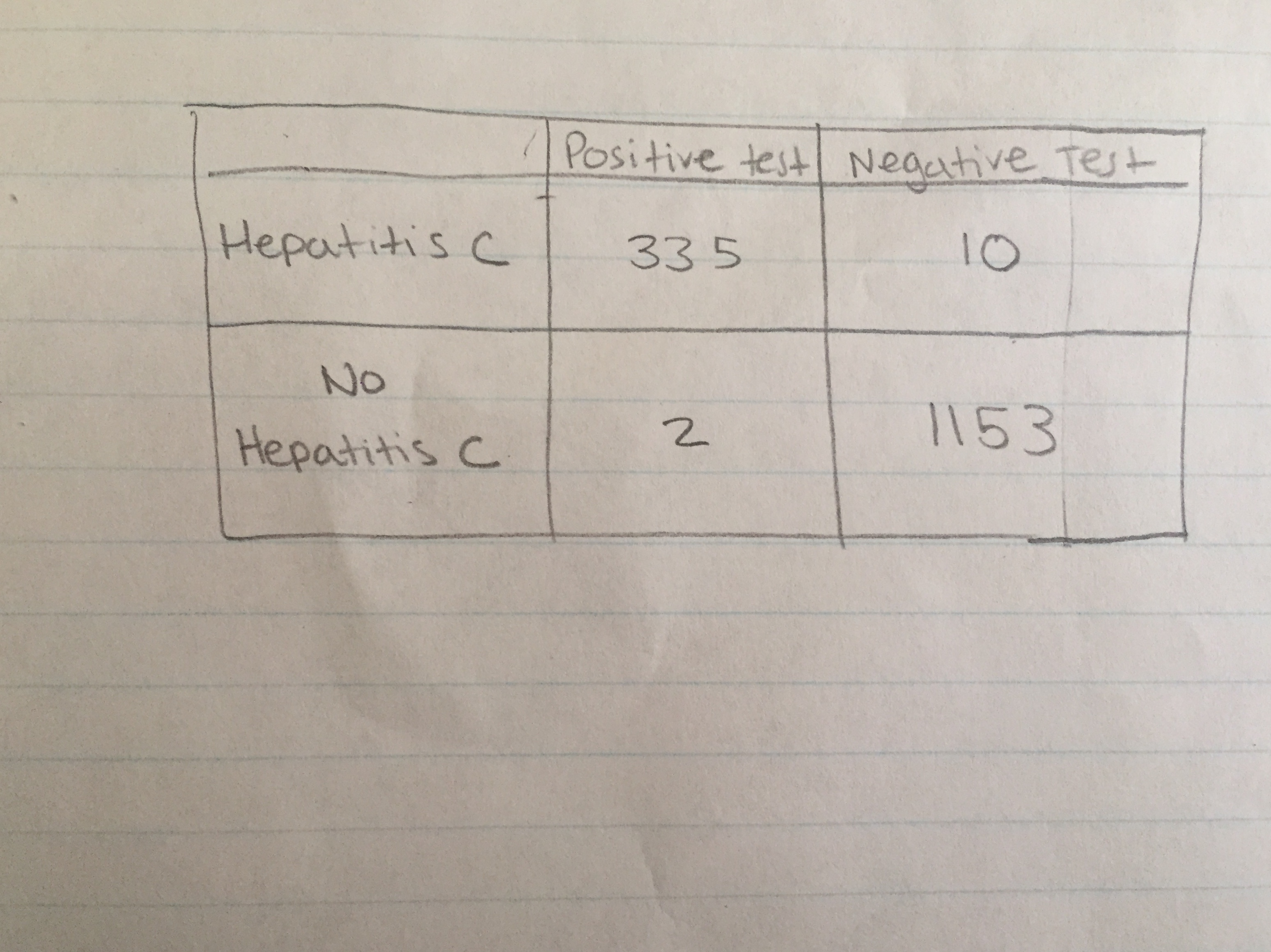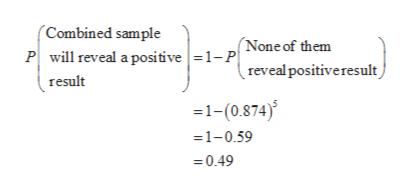# Positive test Neaahive TestHepatitis c33 5No1153Hepatitis cN

Question

23. Composite Drug Test Based on the data in Table 4-1 on page 146, assume that the probability of a randomly selected person testing positive for drug use is 0.126. If drug screening samples are collected from 5 random subjects and combined, find the probability that the combined sample will reveal a positive result. Is that probability low enough so that further testing of the individual samples is rarely necessary?help_outlineImage TranscriptionclosePositive test Neaahive Test Hepatitis c 33 5 No 1153 Hepatitis c N fullscreen
check_circle

Step 1

Obtain the probability that randomly selected person testing negative for drug use:

It is given that probability of a randomly selected person testing positive for drug use is 0.126 and random sample of subject size is 5.

The required probability is,

Step 2
1. Obtain the probability that the combined sample will reveal a positive result:

The required probability is,help_outlineImage Transcriptionclose(Combined sample (None of them Pwil reveal a positive=1-reveal positiveresult ) result 1-(0.874) =1-0.59 0.49 fullscreen
Step 3
1. Identify probability low enough so that further testing of the individual samples is rarely necessary or not:

Here the probability that the combined sample will reveal a positive result is 0.49 and which is greater than 0.05 so the probability is not low.

No, the p...

### Want to see the full answer?

See Solution

#### Want to see this answer and more?

Solutions are written by subject experts who are available 24/7. Questions are typically answered within 1 hour.*

See Solution
*Response times may vary by subject and question.
Tagged in

### Statistics